# 大尺度半分布式WASMOD模型汇流方法改进与应用Improvement and Application of Routing Method for Large Scale Semi-Distributed WASMOD

• 全文下载: PDF(1402KB)    PP.56-66   DOI: 10.12677/JWRR.2019.81006
• 下载量: 187  浏览量: 948

WASMOD水量平衡模型，能满足流域尺度、区域尺度甚至全球尺度的水文模拟，已在全球气候变化影响研究中得到广泛的应用，但其日模型汇流算法没有考虑不同流域地形对流速影响程度的差异。本文以长江流域的湘江、汉江和雅砻江流域为研究对象，建立半分布式日尺度WASMOD模型，通过考虑流域下垫面的影响，研究和改进模型的汇流算法，并比较分析模型汇流方法改进前后的径流模拟效果。结果表明：原模型汇流算法不能适应多种地形情况下的汇流计算，在部分流域计算得到汇流时间相较实际经验值偏长；改进后的模型能合理计算流域汇流时间，从而提高径流模拟精度；汇流算法的改进提高了模型的适用性，改进后的模型能够适应多种地形情况下的径流模拟。

WASMOD, a water balance model, which can meet hydrological simulations at the basin scale, regional scale and even global scale, has been widely used in hydrological response to climate change. But its routing algorithm of daily-scale model does not consider the effect of topography on flow velocity which is variable in different watersheds. This paper establishes a semi-distributed daily-scale WASMOD model in Xiangjiang, Hanjiang and Yalongjiang basins in the Yangtze River basin, improves the model’s routing algorithm by considering the basin underlayer, and compares the model efficiency before and after the improvement. The results show that the original model routing algorithm cannot adapt to the routing calculation under various topography conditions. The delay time calculated in some basins is longer than the empirical value. The modified model can calculate the basin delay time reasonably and improve the model efficiency. The modified model can be adapted to runoff simulation under various topography conditions.

1. 引言

WASMOD (Water and Snow Balance Modeling System)的当前版本由Xu  等人基于NOPEX的流域开发。Widén-Nilsson  等人在原本的WASMOD基础上构建了大尺度水量平衡模型WASMOD-M，Gong  等在WASMOD-M月尺度模型的基础上建立了半分布日尺度WASMOD模型。目前WASMOD模型已有月尺度、日尺度模型，能满足流域尺度、区域尺度甚至全球尺度的水文模拟。

NRF汇流算法可以在所有尺度保留高分辨率地形数据的汇流滞时空间分布信息，在大尺度分布式水文模型的研究方面具有重要的价值。但该算法没有考虑流域的下垫面的影响，不能适应多种流域下垫面情况下的汇流计算。在不同的流域下垫面影响下，同样的坡度对流速的影响程度是不一样的，在本文的研究中发现部分流域计算得到汇流时间相较实际经验值偏长。

2. 研究区和数据

2.1. 研究流域

Table1. Climate and topographic characteristics information for study regional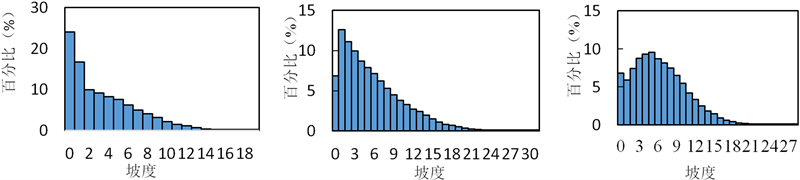(a) 甘溪站控制流域 (b) 雅江站控制流域 (c) 白河站控制流域

Figure 1. Slope-frequency histograms for study area

2.2. 研究数据

WASMOD模型所需气象信息为降雨，温度，和相对湿度。降雨和温度数据分别为国家气象中心发布的中国地面降水日值0.5˚ × 0.5˚格点数据集和中国地面气温日值0.5˚ × 0.5˚格点数据集(http://data.cma.cn/site/showSubject/id/46.html)。站点日径流数据采用水文站实测数据。甘溪水文站采用1999~2009年日径流数据，雅江水文站采用1998~2008年日径流数据，白河水文站采用1980~1990年日径流数据。

3. WASMOD模型及汇流方法

3.1. WASMOD模型介绍

WASMOD是一个水量平衡模型，模型概念简单，参数较少，模型结构图如图2。模型包括降水下渗、蒸发、产流和汇流等四个模块  ，每个过程模块的参数如表2所示  。Figure 2. The concept of the WASMOD model systemTable 2. A list of parameters for WASMOD model system

3.2. NRF汇流方法及问题

NRF算法在流域汇流的模拟过程中，采用式(1)计算产流经过单个格网所需要的时间 ${\epsilon }_{i}$ 。对于每个格网，根据格网流向，可以查找该格网产流流向流域出口的唯一路径，累加产流经过该路径上的所有格网所需的时间即可得到该格网的汇流时间t。

${\epsilon }_{i}=\left\{\begin{array}{cc}\underset{i=1}{\overset{n}{\sum }}\frac{{l}_{i}}{{v}_{45}\cdot \sqrt{\mathrm{tan}\left({c}_{i}\right)}}& {c}_{i}>{c}_{0}\\ \underset{i=1}{\overset{n}{\sum }}\frac{{l}_{i}}{{v}_{45}\cdot \sqrt{\mathrm{tan}\left({c}_{0}\right)}}& {c}_{i}\le {c}_{0}\end{array}$ (1)

${t}_{i}={\sum }_{j=1}^{n}{\epsilon }_{i\left(j\right)}$ (2)

$\frac{\partial Q}{\partial t}=D\frac{{\partial }^{2}Q}{\partial {x}^{2}}-c\frac{\partial Q}{\partial x}$ (3)

$Q\left(0,t\right)={I}_{0}$ (4)

$Q\left(x,0\right)=0,\text{\hspace{0.17em}}\text{\hspace{0.17em}}\text{\hspace{0.17em}}x>0$ (5)

Qc的解为：

${Q}_{c}\left(x,t\right)=\frac{{I}_{0}}{2}\cdot \left[erfc\left(\frac{x-c\cdot t}{\sqrt{4Dt}}\right)+\mathrm{exp}\left(\frac{c\cdot x}{D}\right)\cdot \right]erfc\left(\frac{x+c\cdot t}{\sqrt{4Dt}}\right)$ (6)

${Q}_{24}\left(x,t\right)={Q}_{c}\left(x,t\right)-{Q}_{c}\left(x,t-24\right)$ (7)

$PRF\left({t}_{d}\right)=\underset{{t}_{d}}{\int }{Q}_{24}\left(t\right)\text{d}t$ (8)

$CRF\left({t}_{d}\right)=\frac{1}{n}\underset{i=1}{\overset{n}{\sum }}PR{F}_{j}\left({t}_{d}\right)$ (9)

$NRF\left({t}_{d}\right)=\underset{j=1}{\overset{m}{\sum }}{Q}_{j}\cdot CR{F}_{j}\left({t}_{d}\right)$ (10)

NRF汇流算法，提升了运算精度，简化了运算量，且汇流时间具有与高分辨网格推求出汇流时间同样的精度与稳定性，能够实现高效快速的汇流计算。但公式(1)没有考虑在不同流域下垫面影响下，坡度对流速影响程度是不同的。在部分流域运用式(1)和式(2)计算所得汇流时间过长，明显不符合实际，并导致径流模拟结果纳西效率系数极低。

3.3. NRF汇流方法改进

${\epsilon }_{i}=\left\{\begin{array}{cc}\underset{i=1}{\overset{n}{\sum }}\frac{{l}_{i}}{{v}_{45}\cdot {\left(\mathrm{tan}\left({c}_{i}\right)\right)}^{b}}& {c}_{i}>{c}_{0}\\ \underset{i=1}{\overset{n}{\sum }}\frac{{l}_{i}}{{v}_{45}\cdot {\left(\mathrm{tan}\left({c}_{0}\right)\right)}^{b}}& {c}_{i}\le {c}_{0}\end{array}$ (11)Table 3. A list of parameters for routing module before and after improvement

4. 结果分析和讨论

4.1. 汇流方法改进前后模型径流模拟结果比较Table 4. Initial calibration range of runoff generation parametersTable 5. Initial calibration range of routing parameters before and after improvementTable 6. Calibration and validation of study area before and after improvementTable 7. Optimal parameter of the model after improvement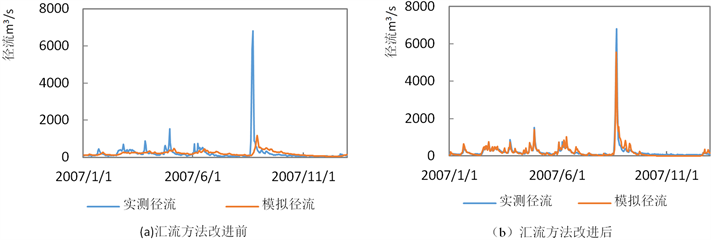Figure 3. Daily observed and simulated discharges at Ganxi basin in 2008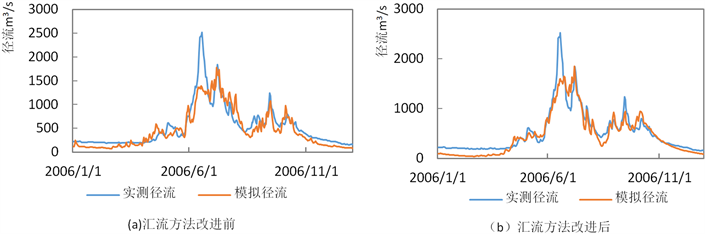Figure 4. Daily observed and simulated discharges at Yajiang catchment in 2006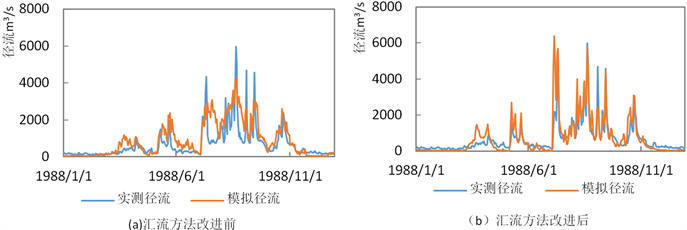Figure 5. Daily observed and simulated discharges at Beihe catchment in 1988

4.2. 汇流时间变化分析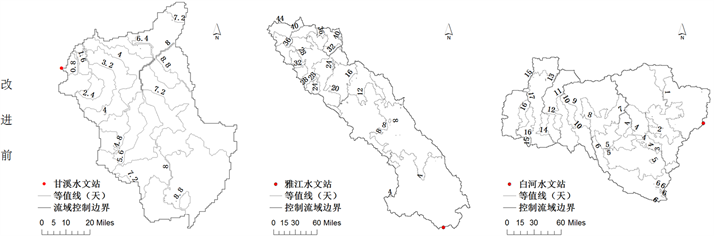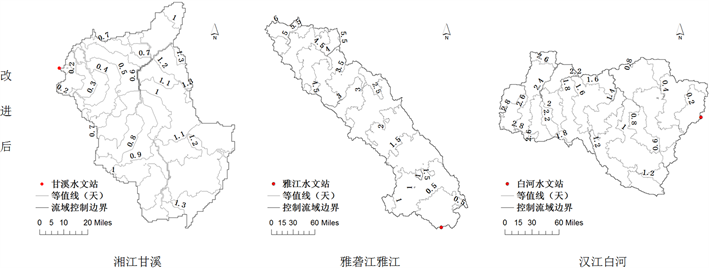Figure 6. Distribution of delays times for study areas

5. 结论

  ARNELL, N. W., GOSLING, S. N. The impacts of climate change on river flood risk at the global scale. Climatic Change, 2016, 134(3): 387-401. https://doi.org/10.1007/s10584-014-1084-5  许崇育, 夏军. 大尺度水文模型的发展现状以及与气候模型耦合的可能性、挑战和展望[C]//中国自然资源学会. 中国自然资源学会2011年学术年会: 2011年卷. XU Chongyu, XIA Jun. The development of large-scale hydrological models and the possibility, challenge and prospect of coupling them with climate models. 2011 annual conference of China natural resources association, 2011. (in Chi-nese)  DÖLL, P., KASPAR, F. and LEHNER, B. A global hydrological model for deriving water availability indicators: Model tuning and validation. Journal of Hydrology, 2003, 270(1): 105-134. https://doi.org/10.1016/S0022-1694(02)00283-4  LIANG, X., LETTENMAIER, D. P. and WOOD, E. F. One-dimensional statistical dynamic representation of subgrid spatial variability of precipitation in the two-layer variable infiltration capacity model. Journal of Geophysical Research Atmospheres, 1996, 101(D16): 21403-21422.  VÖRÖSMARTY, C. J., FEDERER, C. A. and SCHLOSS, A. L. Potential evaporation functions compared on US watersheds: Possible implications for global-scale water balance and terrestrial ecosystem modeling. Journal of Hydrology, 1998, 207(3-4): 147-169.  ARNELL, N. W. A simple water balance model for the simulation of streamflow over a large geographic domain. Journal of Hydrology, 1999, 217(3): 314-335. https://doi.org/10.1016/S0022-1694(99)00023-2  XU, C. Y., SEIBERT, J. and HALLDIN, S. Regional water balance modelling in the NOPEX area: Development and application of monthly water balance models. Journal of Hydrology, 1996, 180(1-4): 211-236.  WIDÉN-NILSSON, E., GONG, L., HALLDIN, S., et al. Model performance and parameter behavior for varying time aggregations and evaluation criteria in the WASMOD-M global water balance model. Water Resources Research, 2009, 45(5): 5418. https://doi.org/10.1029/2007WR006695  GONG, WIDÉN-NILSSON, HALLDIN, et al. Large-scale runoff routing with an aggregated network-response function. Journal of Hydrology, 2009, 368(1): 237-250. https://doi.org/10.1016/j.jhydrol.2009.02.007  XU, C. Y. Application of water balance models to different climatic regions in China for water resources assessment. Water Resources Management, 1997, 11(1): 51-67. https://doi.org/10.1023/A:1007945406728  WIDÉN-NILSSON, E., HALLDIN, S. and XU, C. Y. Global wa-ter-balance modelling with WASMOD-M: Parameter estimation and regionalisation. Journal of Hydrology, 2007, 340(1): 105-118. https://doi.org/10.1016/j.jhydrol.2007.04.002  EREGNO, F. E., XU, C. Y. and KITTERØD, N. O. Modeling hydrological impacts of climate change in different climatic zones. International Journal of Climate Change Strategies & Management, 2013, 5(3): 344-365. https://doi.org/10.1108/IJCCSM-04-2012-0024  KIZZA, M., GUERRERO, J. L., RODHE, A., et al. Modelling catchment inflows into Lake Victoria: Regionalisation of the parameters of a conceptual water balance model. Hydrology Research, 2013, 44(5): 789-808. https://doi.org/10.2166/nh.2012.152  李占玲, 徐宗学, 周训. WASMOD水文模拟残差统计特征检验[J]. 水利水电科技进展, 2013, 33(1): 13-17. LI Zhanling, XU Zongxue and ZHOU Xun. Check of statistical features of model residuals of WASMOD. Advances in Science and Technology of Water Resources, 2013(1): 13-17.  LI, L., NGONGONDO, C. S., GONG, L., et al. Comparison of the global TRMM and WFD precipitation datasets in driving a large-scale hydrological model in Southern Africa. Hydrology Research, 2013, 44(5): 770-788. https://doi.org/10.2166/nh.2012.175  熊立华, 彭定志. 基于数字高程模型的等流时线推求与应用[J]. 武汉大学学报(工学版), 2003, 36(3): 1-3. XIONG Lihua, PENG Dingzhi. Derivation of DEM-based isochrones of watersheds. Journal of Wuhan University of Hydraulic & Electric Engineering, 2003, 36(3): 1-3.  陈洋波. 基于HYDRO1K的数字水文分析方法及实例[J]. 人民长江, 2002, 33(9): 52-54. CHEN Yangbo. Digital hydrological analysis methods and examples based on HYDRO1K. Yangtze River, 2002, 33(9): 52-54. (in Chinese)  XU, C. Y., SINGH, V. P. and FREVERT, D. WASMOD: The water and snow balance modeling system. 2002.  GONG, L. Large-scale runoff generation and routing: Efficient parameterization using high-resolution topography and hydrography. PhD. Thesis, Uppsala University, Uppsala, 2010.